# Problem 15 15 of 21 > A Review Constants Using 2.7 K resistors and ideal op...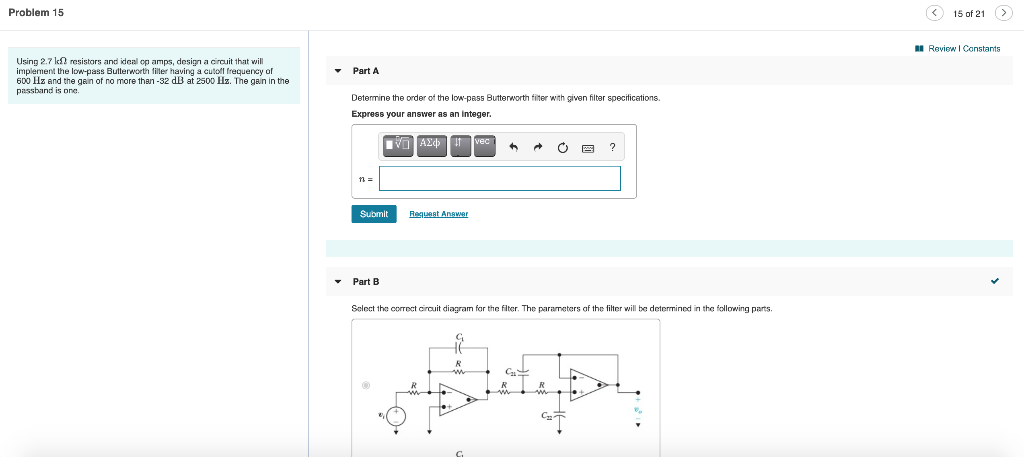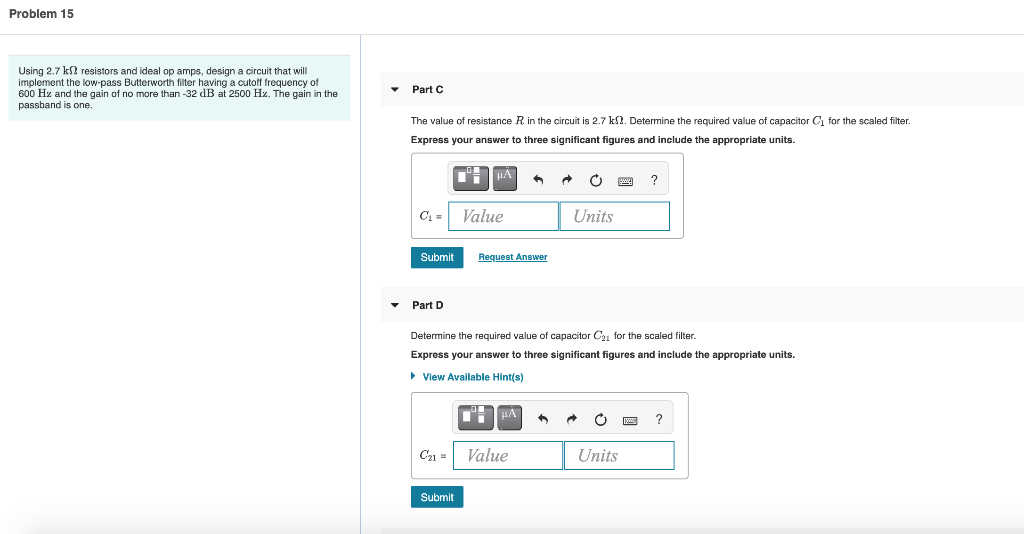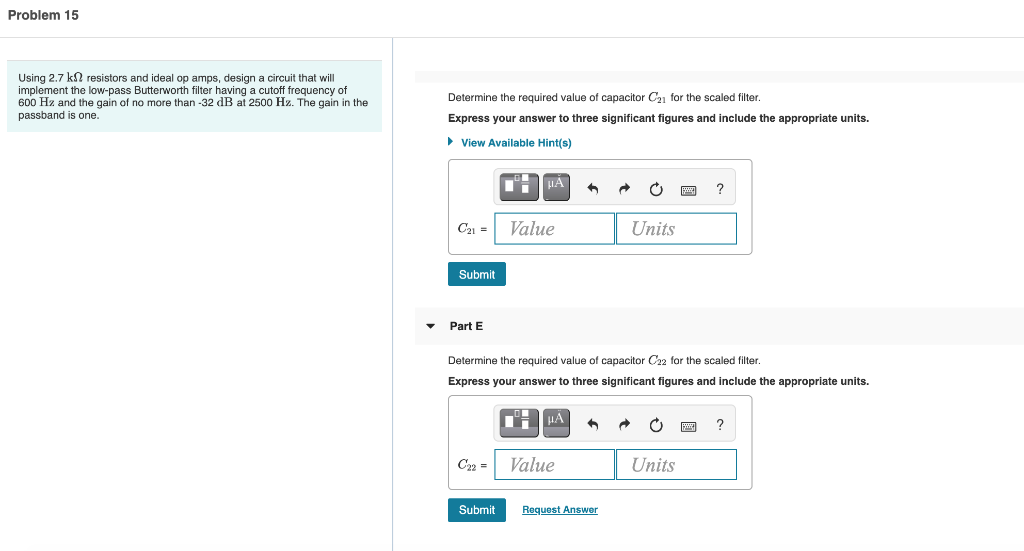Problem 15 15 of 21 > A Review Constants Using 2.7 K resistors and ideal op amps, design a circuit that will implement the low-pass Butterworth filter having a cutoll frequency of 600 Hz and the gain of no more than-32 dB at 2500 Hz. The gain in the passband is one Part A Determine the order of the low-pass Butterworth filter with given filter specifications. Express your answer as an integer. IVO AX t ? 11 = Submit Request Answer - Part B Select the correct circuit diagram for the filter. The parameters of the filter will be determined in the following parts. G.
Problem 15 Using 2.7 k resistors and ideal op amps, design a circuit that will implement the low-pass Butterworth filter having a cutoff frequency of 600 Hz and the gain of no more than -32 dB at 2500 Hz. The gain in the passband is one. Part The value of resistance R in the circuit is 2.7 k 2. Determine the required value of capacitor for the scaled filter. Express your answer three significant figures and include the appropriate units. HA C= Value Units Submit Request Answer Part D Determine the required value of capacitor C2 for the scaled filter. Express your answer three significant figures and include the appropriate units. View Available Hint(s) ? C21 = Value Units Submit
Problem 15 Using 2.7 k resistors and ideal op amps, design a circuit that will implement the low-pass Butterworth filter having a cutoff frequency of 600 Hz and the gain of no more than -32 dB at 2500 Hz. The gain in the passband is one. Determine the required value of capacitor C2 for the scaled filter. Express your answer to three significant figures and include the appropriate units. ► View Available Hint(s) μΑ h ? C21 = Value Units Submit Part E Determine the required value of capacitor C22 for the scaled filter. Express your answer to three significant figures and include the appropriate units. HA ? C22 = Value Units Submit Request Answer

Hi friend...I hope this helps you...for any doubt feel free to comment... thankyou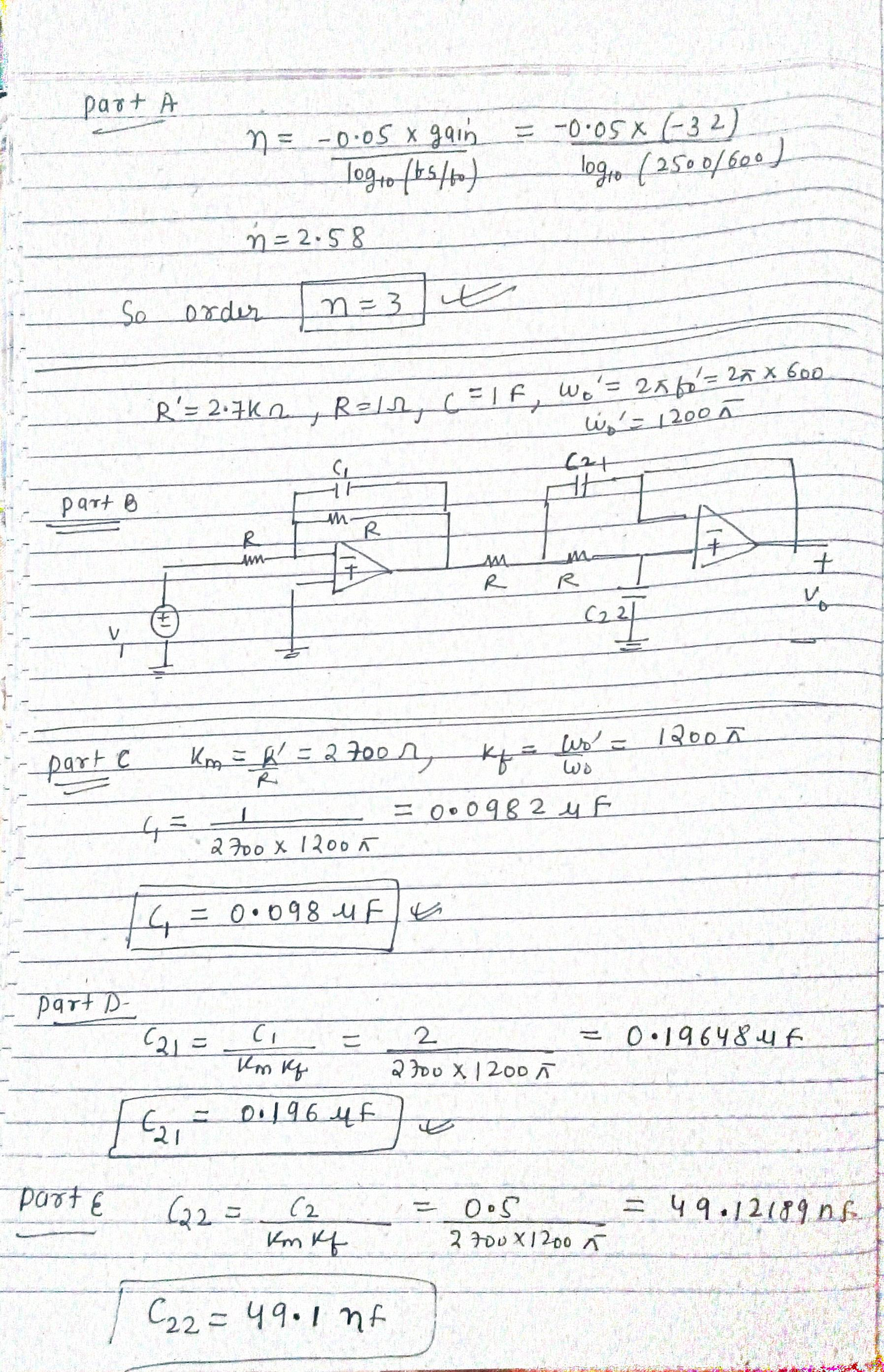#### Earn Coin

Coins can be redeemed for fabulous gifts.

Similar Homework Help Questions
• ### Using C 210 nF capacitor and other required capacitors, resistors, and ideal op amps, design a...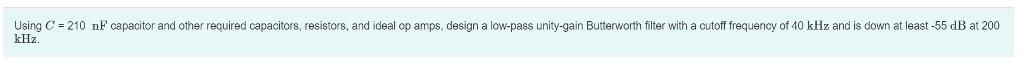Using C 210 nF capacitor and other required capacitors, resistors, and ideal op amps, design a low-pass unity-gain Butterworth filter with a cutoff frequency of 40 kHz and is down at least-55 dB at 200 kHz.

• ### Problem 4. (6 marks) You are required to design a third-order Butterworth bandpass filter using ideal...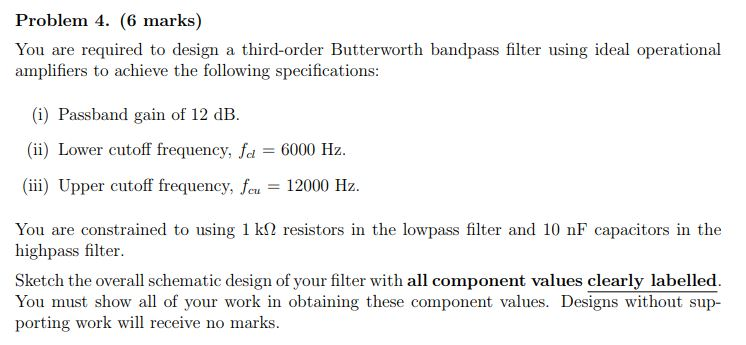Problem 4. (6 marks) You are required to design a third-order Butterworth bandpass filter using ideal operational (6) Passband gain of 12 dB. (i) Lower cutoff frequency, f 6000 Hz. (ii) Upper cutoff frequency, u 12000 Hz. You are constrained to using 1 k? resistors in the lowpass filter and 10 nF capacitors in the highpass filter. Sketch the overall schematic design of your filter with all component values clearly labelled. You must show all of your work in obtaining...

• ### Part B Using C = 26 nF capacitors, design an active broadband first-order bandreject filter with...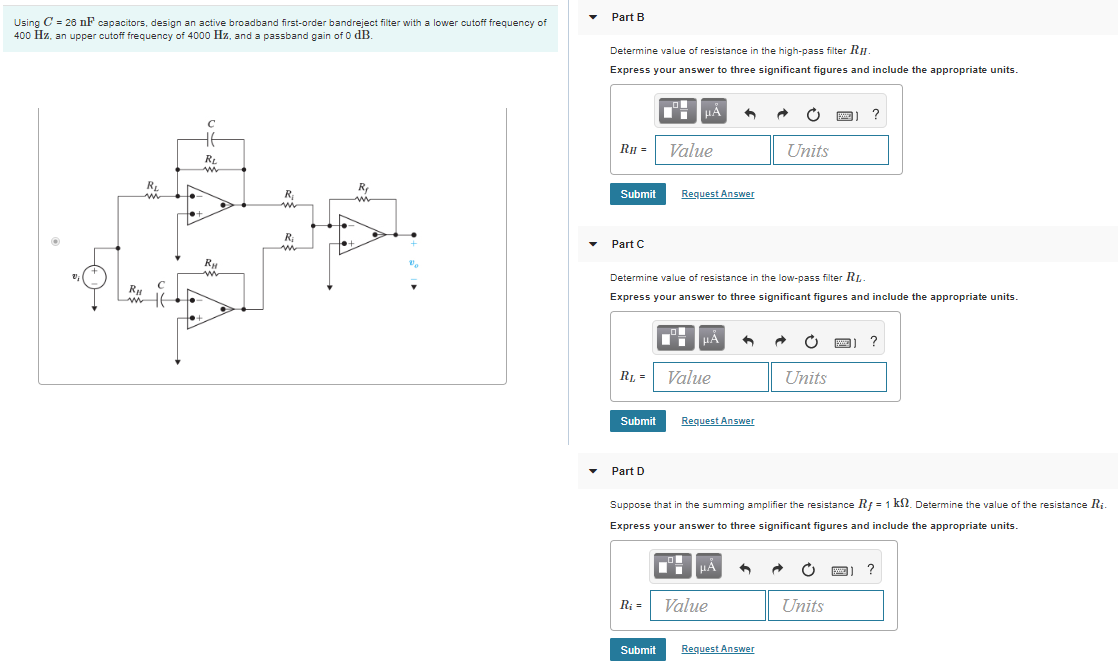Part B Using C = 26 nF capacitors, design an active broadband first-order bandreject filter with a lower cutoff frequency of 400 Hz, an upper cutoff frequency of 4000 Hz, and a passband gain of 0 dB. Determine value of resistance in the high-pass filter RH. Express your answer to three significant figures and include the appropriate units. RH = Value Units Submit Request Answer Part C Determine value of resistance in the low-pass filter RL Express your answer to...

• ### Learning Goal: To analyze and design a passive, first-order low- pass filter using a series RL...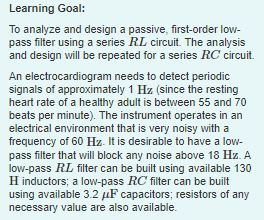Learning Goal: To analyze and design a passive, first-order low- pass filter using a series RL circuit. The analysis and design will be repeated for a series RC circuit. An electrocardiogram needs to detect periodic signals of approximately 1 Hz (since the resting heart rate of a healthy adult is between 55 and 70 beats per minute). The instrument operates in an electrical environment that is very noisy with a frequency of 60 Hz. It is desirable to have a...

• ### 53. A 2- order normalized Butterworth filter can be improved by using a so-called Chebeyshev filter...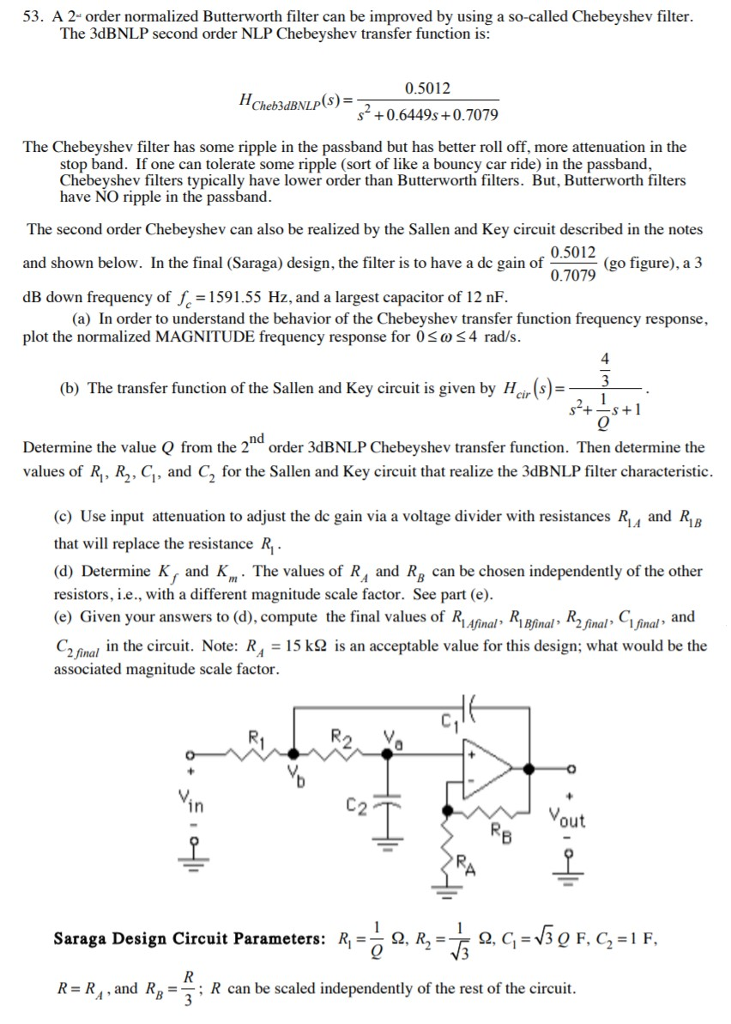53. A 2- order normalized Butterworth filter can be improved by using a so-called Chebeyshev filter The 3dBNLP second order NLP Chebeyshev transfer function is: 0.5012 2 +0.6449s+0.7079 Cheb3dBNLP(s) The Chebeyshev filter has some ripple in the passband but has better roll off, more attenuation in the stop band. If one can tolerate some ripple (sort of like a bouncy car ride) in the passband Chebeyshev filters typically have lower order than Butterworth filters. But, Butterworth filters have NO ripple...

• ### Learning Goal: To analyze and design a passive, first-order low-pass filter using a series RL circuit....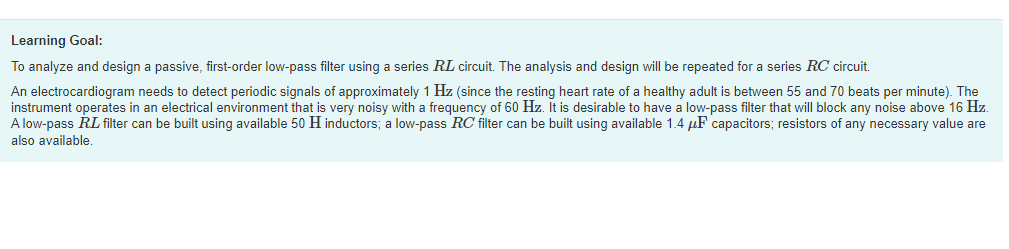Learning Goal: To analyze and design a passive, first-order low-pass filter using a series RL circuit. The analysis and design will be repeated for a series RC circuit. An electrocardiogram needs to detect periodic signals of approximately 1 Hz (since the resting heart rate of a healthy adult is between 55 and 70 beats per minute). The instrument operates in an electrical environment that is very noisy with a frequency of 60 Hz. It is desirable to have a low-pass...

• ### We were unable to transcribe this image5. (a) Assume Ri = 122, R412. L = 2...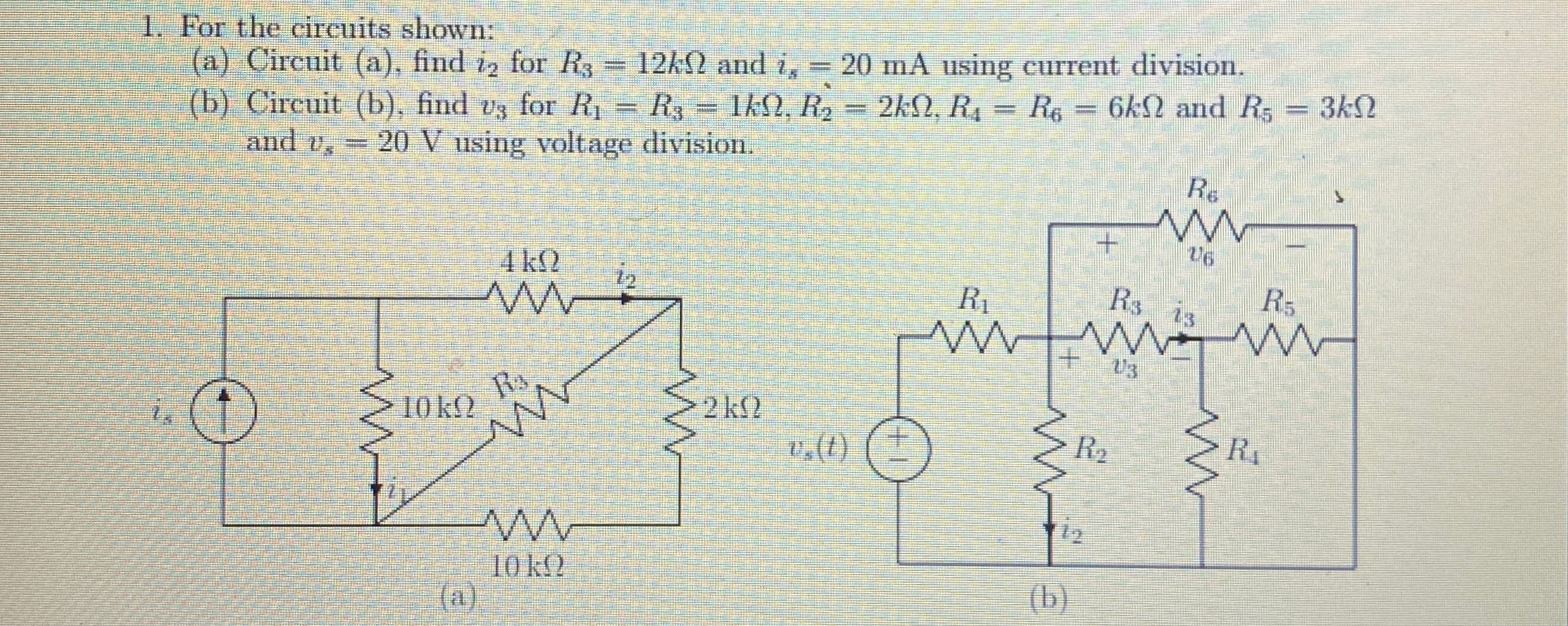We were unable to transcribe this image5. (a) Assume Ri = 122, R412. L = 2 H, and C F for the following circuit. Use Laplace transforms and Thevenin's theorem to find vo(). 12u(0 V ) to(SR Tb) Using 1 k22 resistors and op amps, design a high-pass unity gain Butterworth filter With a cutoff frequency of 8 kHz and and is down at least 48 dB at 32 kHz. Draw your circuit diagram and label all the component values.

• ### Problem 2: In the circuit shown assume the Op-Amp is ideal. A) Find vo as a...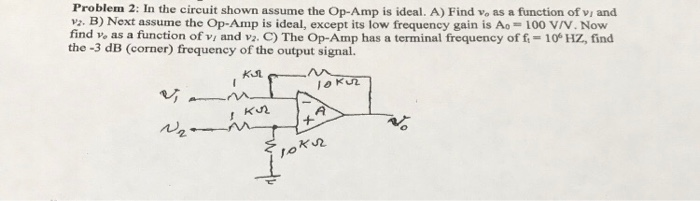Problem 2: In the circuit shown assume the Op-Amp is ideal. A) Find vo as a function of vi and vs. B) Next assume the Op-Amp is ideal, except its low frequency gain is Ao= 100 V/V. Now find vo as a function of vi and v2. C) The Op-Amp has a terminal frequency of fi 10° Hz, find the -3 dB (corner) frequency of the output signal w2 10K

• ### 10 Design a low pass filter using a resistor R and a capacitor C of 0.2uF....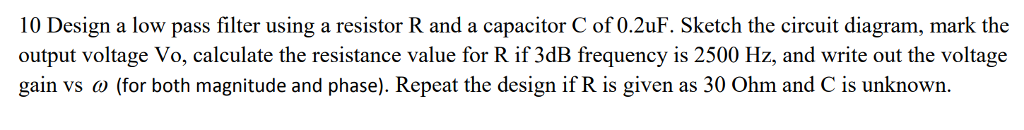10 Design a low pass filter using a resistor R and a capacitor C of 0.2uF. Sketch the circuit diagram, mark thoe output voltage Vo, calculate the resistance value for R if 3dB frequency is 2500 Hz, and write out the voltage gain vs ω (for both magnitude and phase). Repeat the design if R is given as 30 Ohm and C is unknown.

• ### Student number: 40252516 since its needed to solve the questions. my student number is 40252516 its...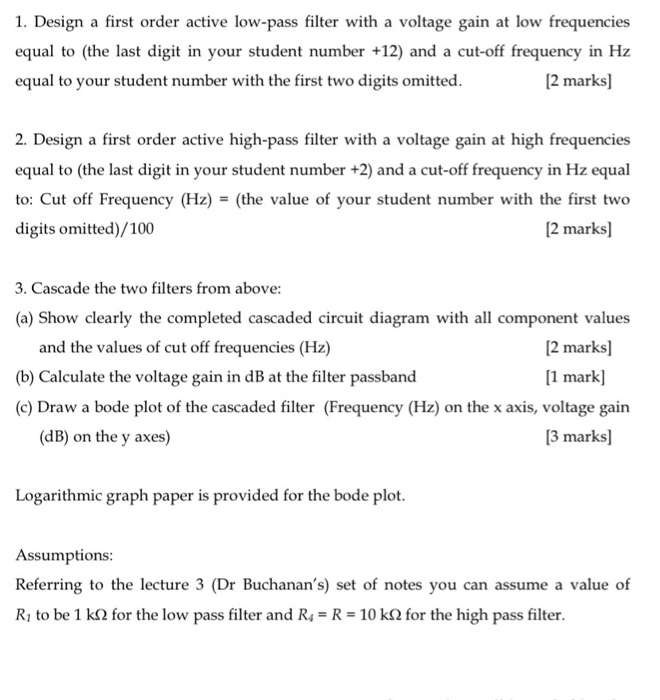Student number: 40252516 since its needed to solve the questions. my student number is 40252516 its needed to solve the questions. 1. Design a first order active low-pass filter with a voltage gain at low frequencies equal to (the last digit in your student number +12) and a cut-off frequency in Hz equal to your student number with the first two digits omitted. [2 marks] 2. Design a first order active high-pass filter with a voltage gain at high frequencies...